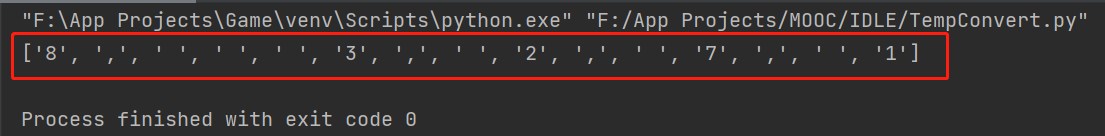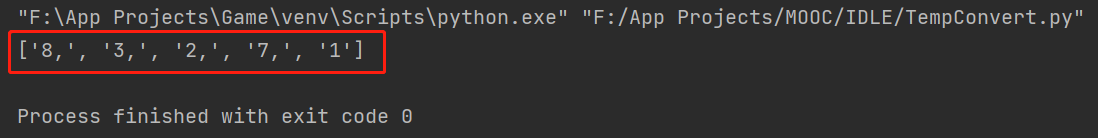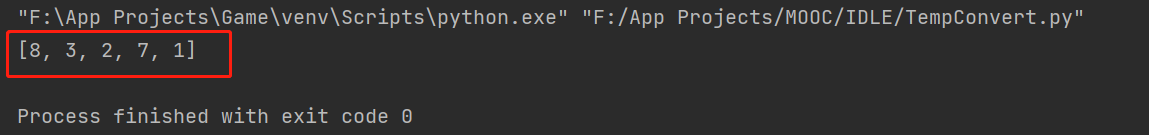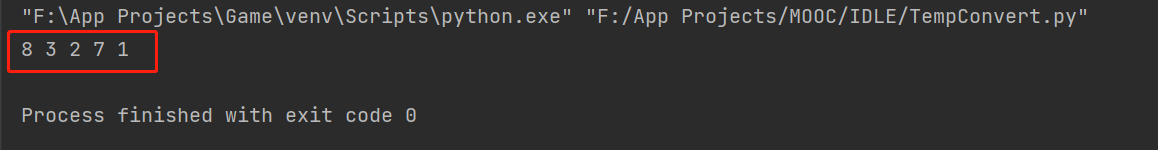### Python字符串与列表之间的转换方法

1. 利用 list() 方法，默认把空格、逗号等所有符号在内的每一个字符串元素逐个打印，分别作为列表的每个元素：

``s = '8,   3, 2, 7, 1'l = list(s)print(l)``2. 利用 split() 方法，把字符串以某种方式变成列表，如没有参数，默认为以空格区分每一个字符串元素并依次转换为列表元素：

``s = '8,   3, 2, 7, 1'l = s.split()print(l)``1. str() 方法

``l = [8,   3, 2, 7, 1]s = str(l)print(s)``str() 在列表外层加上了一个引号‘’，但不显示在控制台。

2. join() 方法：按照空格分割字符串元素。

``l = ['8',   '3', '2', '7', '1']s = ' '.join(l)print(s)``注意，使用join()时，列表中的元素必须是字符串格式，表现为元素必须加引号，不能为整数类型或其他类型。